• 层次分析法中判断矩阵的填写方法、一致性检验的步骤、以及根据判断矩阵计算权重的方法

目录：

准则层判断矩阵怎么填写
方案层判断矩阵怎么填写
关于判断矩阵和一致矩阵的知识点补充
一致性检验的步骤
怎样通过判断矩阵去计算权重（三种方法），及相应的代码示例

准则层判断矩阵的填写：
填写准则层判断矩阵的目的是确定各准则（指标）所占的比重，填写好层次分析表的指标权重列，例如在选择最佳旅游地问题的指标景色、花费、居住、饮食、交通各自占比是多少，后续可以通过这些指标占比计算出每一个可选方案的总分。填表的方法是依据标度表，两两比较指标的重要程度，只需要比较10次就可以完成准则层判断矩阵的填写

方案层判断矩阵的填写
填写方案层判断矩阵的目的是给出，对于某一特定指标，它在各个可选方案的具体得分是多少，也就是给出层次分析表的每一横行的数据。方法是依据标度表，填写好判断矩阵。有几个评价指标，就需要填多少此方案层判断矩阵。知识点补充：
判断矩阵（正互反矩阵）

首先判断矩阵一定是一个方阵
判断矩阵每一个数据 Aij表示与指标 j相比 i的重要程度
当 i=j 时，两个指标相同，因此同等重要，记为1，因此判断矩阵的对角线元素为1
每一个元素均大于零，且 Aij * Aji=1

在层次分析法中，我们构造的矩阵的均为判断矩阵
一致矩阵

矩阵首先满足判断矩阵的所有特点
若判断矩阵满足 Aij * Ajk = Aik,直观的看就是矩阵的各行（各列）成倍数关系

注意点：在使用判断矩阵求权重之前，必须对其进行一致性检验

一致性检验的步骤：
第一步：计算一致性指标CI
$CI\,\,=\,\,\frac{\lambda _{\max}-n}{n-1}$
第二步：查找对应的平均随机一致性指标RI第三步：计算一致性比例CR
$CR\,\,=\,\,\frac{CI}{RI}$
判断：如果CR<0.1,则可认为判断举证的一致性可以接受；否则需要对判断矩阵进行修改
一致性检验的MATLAB代码如下：
disp('请输入判断矩阵A')
A=input('A=');
[n,n] = size(A);
[V,D] = eig(A);%求出矩阵A的特征值和特征向量
Max_eig = max(max(D));%找到矩阵A的最大特征值
% 下面是计算一致性比例CR的环节 %
CI = (Max_eig - n) / (n-1);
RI=[0 0.0001 0.52 0.89 1.12 1.26 1.36 1.41 1.46 1.49 1.52 1.54 1.56 1.58 1.59];
%注意哦，这里的RI最多支持 n = 15
% 这里n=2时，一定是一致矩阵，所以CI = 0，我们为了避免分母为0，将这里的第二个元素改为了很接近0的正数
CR=CI/RI(n);
disp('一致性指标CI=');disp(CI);
disp('一致性比例CR=');disp(CR);
if CR<0.10
disp('因为CR<0.10，所以该判断矩阵A的一致性可以接受!');
else
disp('注意：CR >= 0.10，因此该判断矩阵A需要进行修改!');
end



通过判断矩阵求权重
方法一、算数平均法求权重
第一步：将判断矩阵按照列归一化（每一个元素除以器所在列的和）
第二步：将归一化的各列相加（按行求和）
第三步：将相加后得到的向量中的每个元素除以n即可得到权重向量
具体数学表达：
假设判断矩阵为下面这个矩阵A：
$A=\left[ \begin{matrix} a_{11}& a_{12}& \cdots& a_{1n}\\ a_{21}& a_{22}& \cdots& a_{2n}\\ \vdots& \vdots& \ddots& \vdots\\ a_{n1}& a_{n2}& \cdots& a_{nn}\\ \end{matrix} \right]$
那么算数平均法求得的权重向量为：
$w_i=\frac{1}{n}\sum_{j=1}^n{\frac{a_{ij}}{\sum_{k=1}^n{a_{kj}}}}\,\,$
MATLAB代码如下：
disp('请输入判断矩阵A')
A=input('A=');
[n,n] = size(A);

Sum_A = sum(A);   %sum函数默认是对矩阵的每一列进行累加，即按行求和
SUM_A = repmat(Sum_A,n,1);    %将Sum_A这个行向量，重复n行，重复一列
Stand_A = A ./ SUM_A;         %将矩阵A归一化，即每一个元素除以其所在列的和

disp('算术平均法求权重的结果为：');
disp(sum(Stand_A,2)./n)      %把归一化的矩阵的每一行累加，然后除以n,得到权重

方法二、几何平均法求权重
第一步：将A元素按照行相乘得到一个新的列向量
第二步：将新的列向量的每个分量开n次方
第三步：对该列向量进行归一化即可得到权重向量
假设判断矩阵为下面这个矩阵A：
$A=\left[ \begin{matrix} a_{11}& a_{12}& \cdots& a_{1n}\\ a_{21}& a_{22}& \cdots& a_{2n}\\ \vdots& \vdots& \ddots& \vdots\\ a_{n1}& a_{n2}& \cdots& a_{nn}\\ \end{matrix} \right]$
那么几何平均法求得的权重向量为：
$w_i=\frac{\left( \prod_{j=1}^n{a_{ij}} \right) ^{\frac{1}{n}}}{\sum_{k=1}^n{\left( \prod_{j=1}^n{a_{kj}} \right) ^{\frac{1}{n}}}},\left( i=1,2,…\text{，}n \right)$
注意：每一种方法求得的权重和应该为1，由于四舍五入导致的误差可以忽略，一般结果保留四位小数
MATLAB代码如下：
disp('请输入判断矩阵A')
A=input('A=');
[n,n] = size(A);  %获得矩阵A的行和列的大小

Prduct_A = prod(A,2);       %把矩阵A的每一行累乘，即按照列累乘
Prduct_n_A = Prduct_A .^ (1/n);   %将新的列向量的每个分量开n次方
disp('几何平均法求权重的结果为：');
disp(Prduct_n_A ./ sum(Prduct_n_A))  %对该列向量进行归一化即可得到权重向量

方法三、特征值法求权重（常用）
知识点提醒：一致矩阵有一个特征值为n,其余特征值均为0，并且当矩阵的特征值为n时，其对应的特征向量为
$k\left[ \frac{1}{a_{11}},\frac{1}{a_{12}},…,\frac{1}{a_{1n}} \right] ^T,\left( k\ne 0 \right)$
第一步：求出矩阵A的最大特征值和以及其对应的特征向量
第二步：对求出的特征向量进行归一化即可得到所求的权重
MATLAB代码如下：
disp('请输入判断矩阵A')
A=input('A=');

%求矩阵A的全部特征值，构成对角阵D，求A的特征向量构成V的列向量（V的每一列都是D中与之相同列的特征值的特征向量）
[V,D] = eig(A);
Max_eig = max(max(D));   %求出矩阵A的最大的特征值
[r,c]=find(D == Max_eig , 1);  %返回最大特征值所在的行和列，其中C记录所在列
disp('特征值法求权重的结果为：');
disp( V(:,c) ./ sum(V(:,c)) )  %对最大特征值对应的特征向量进行归一化处理

友情提示：在比赛当中，建议三种方法全部列出来，但仅适用特征值法求得的权重结果进行计算

更多有关与层次分析法的经典获奖论文，以及建模论文模板等资料关注公众号，回复，“层次分析法”，即可免费领取！！！展开全文• 决策者利用一定的标度对属性进行两两比较，并构造判断矩阵，然后按一定的排序方法计算判断矩阵的排序向量，从而获得属性权重，最后在根据各种算子进行多属性群决策。 （一）模糊互补判断矩阵排序法 1.加型模糊互补...
决策者利用一定的标度对属性进行两两比较，并构造判断矩阵，然后按一定的排序方法计算判断矩阵的排序向量，从而获得属性权重，最后在根据各种算子进行多属性群决策。
（一）模糊互补判断矩阵排序法
1.加型模糊互补判断矩阵排序的中转法
2.乘型模糊互补判断矩阵排序的和积法
（二）模糊互补判断矩阵的最优化排序方法

加型模糊互补判断矩阵排序的最小方差法
乘型模糊互补判断矩阵排序的最小平方法
模糊互补判断矩阵排序的幂法

（一）模糊互补判断矩阵排序法
4. 加型模糊互补判断矩阵排序的中转法
判断矩阵的标度和含义如下表所示：
标度	含义
0.1	极端不重要（前比后）
0.3	明显不重要
0.5	同等重要
0.7	明显重要
0.9	极端重要
按上述标度构成判断矩阵，bii=0.5，也满足其他条件。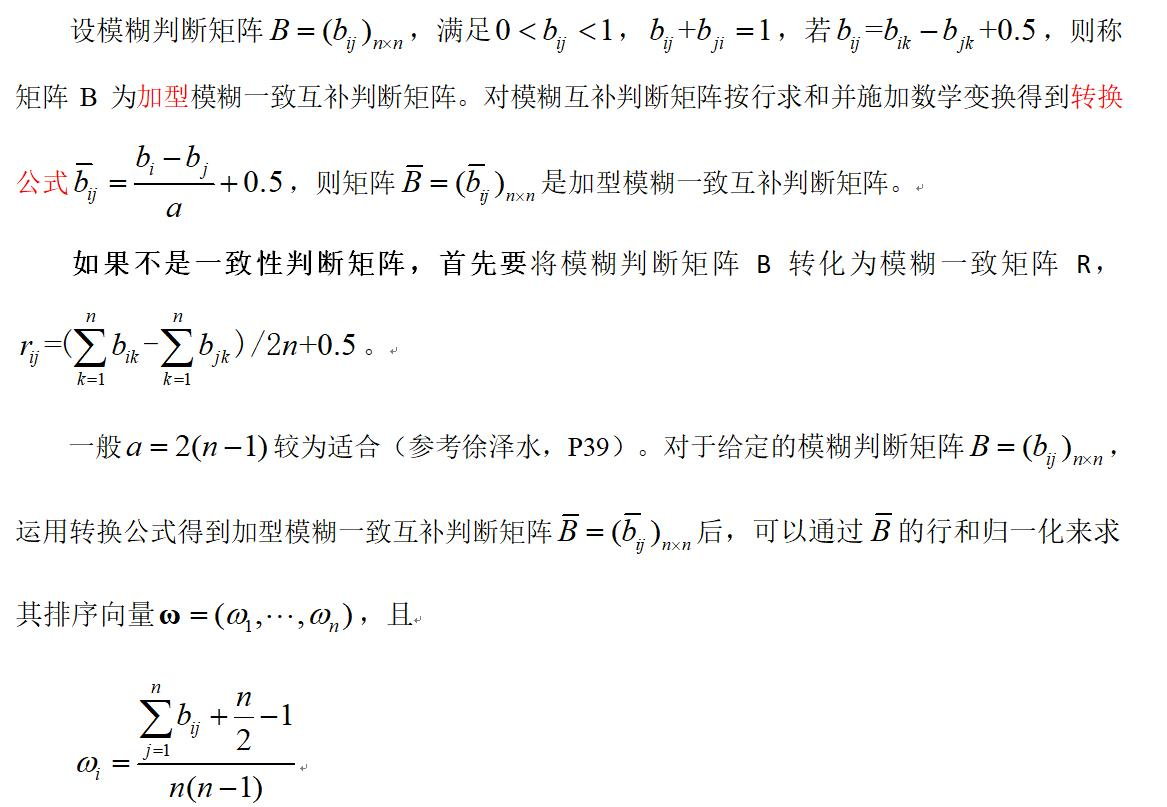此方法称为模糊互补判断矩阵排序的中转法（MTM）。
特点：该方法得到的排序向量的分量之间的差异较小，有时不易区分。
2.乘型模糊互补判断矩阵排序的和积法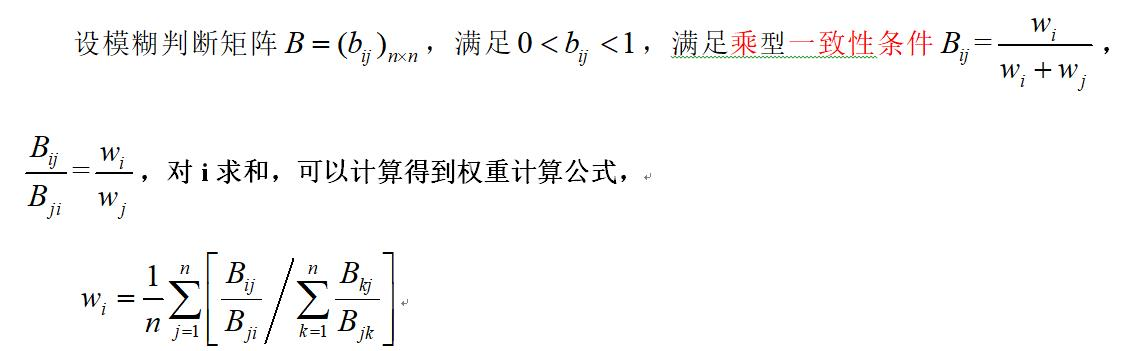特点：该方法得到的排序向量的分量之间的差异较小，有时不易区分。
（二）模糊互补判断矩阵的最优化排序方法
上面介绍的模糊判断矩阵的排序方法前提是所给判断矩阵满足一致性要求，但是并不是所有专家所给的模糊互补判断矩阵都是满足一致性的，甚至可以说更多的是不满足一致性要求的，为此才提出了基于一致性的模糊判断矩阵的最优化排序权重的确定方法。以下简要介绍相关的排序方法。

加型模糊互补判断矩阵排序的最小方差法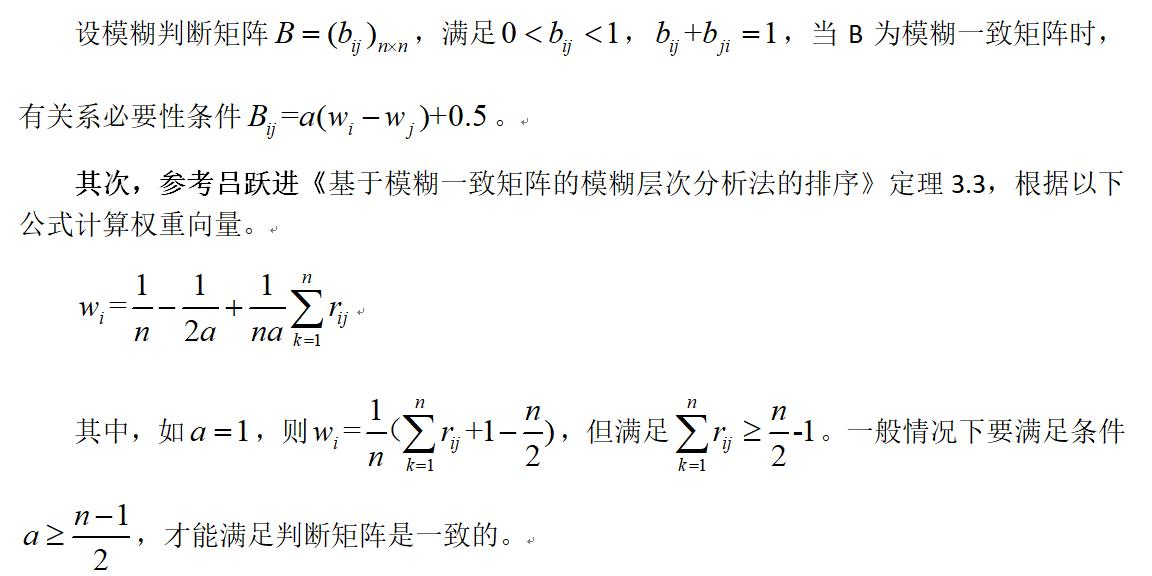乘型模糊互补判断矩阵排序的最小平方法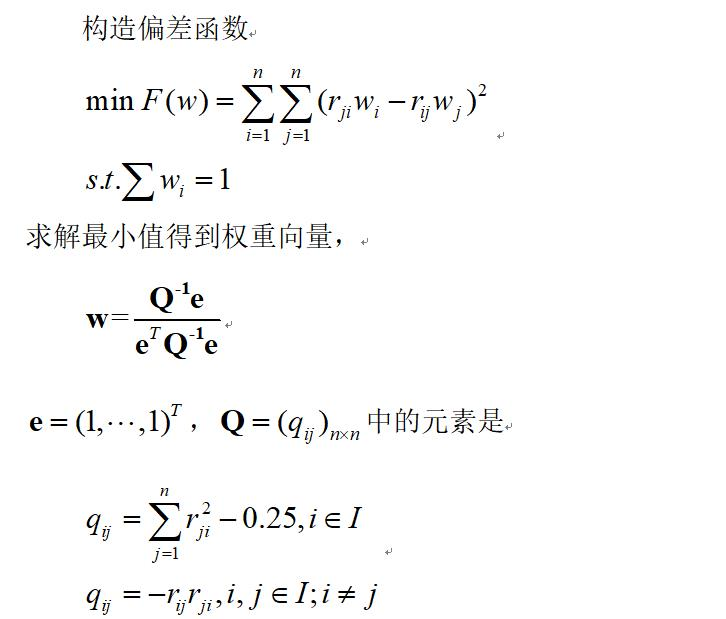3.模糊互补判断矩阵排序的幂法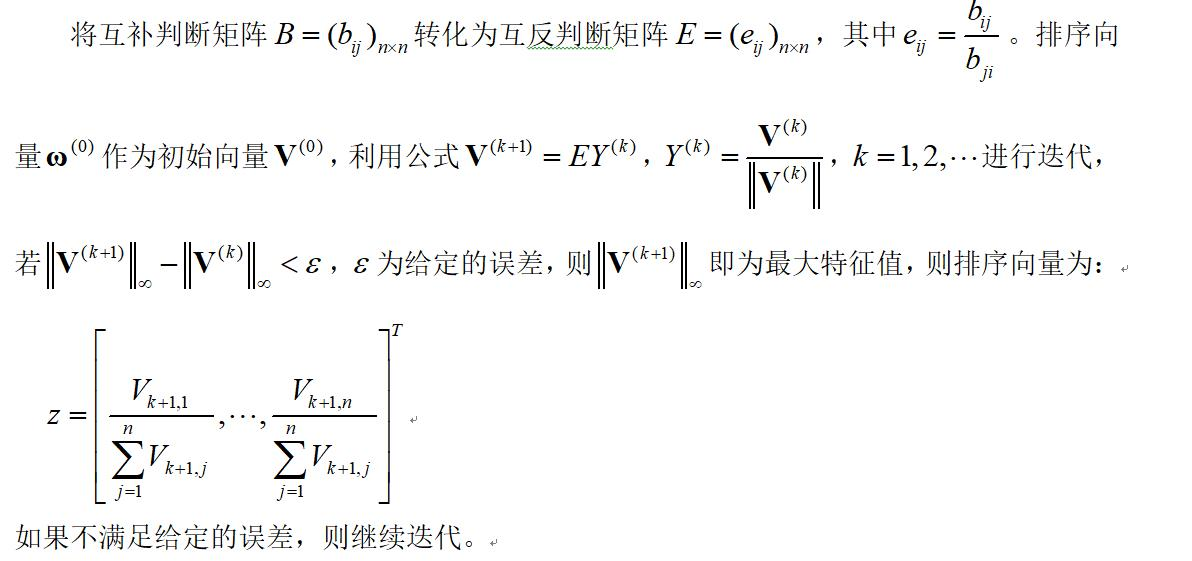（三）实例
1.决策矩阵
有一规范化后的决策矩阵A，如下表所示，
u1	u2	u3	u4	u5
x1	1	0.776	0.828	1	0.412
x2	0.516	1	1	0.296	0.480
x3	0.990	0.627	0.669	1	0.508
x4	0.535	0.784	0.970	0.360	0.678
x5	0.788	0.560	0.578	0.589	1

决策者根据0.1-0.9互补标度对属性进行了两两比较，给出模糊互补判断矩阵B。
0.5	0.6	0.5	0.9	0.7
0.4	0.5	0.4	0.7	0.5
0.5	0.6	0.5	0.7	0.2
0.1	0.3	0.3	0.5	0.1
0.3	0.5	0.8	0.9	0.5
2.算法步骤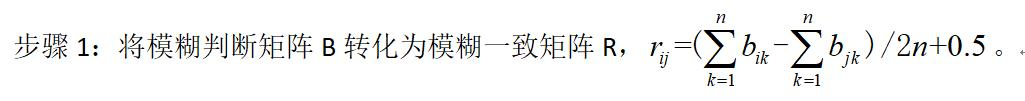步骤2：分别用模糊互补判断矩阵排序的中转法、最小方差法和幂法计算属性的权重向量。
步骤3：再用属性的权重向量，计算各方案综合属性值
步骤4：对方案进行排序。
3.matlab程序
clear;clc;
A=[1	    0.776	0.828	1
0.516	1	    1	    0.296
0.990	0.627	0.669	1
0.535	0.784	0.970	0.360
0.788	0.560	0.578	0.589]

B=[0.5	0.6	0.5	0.9	0.7
0.4	0.5	0.4	0.7	0.5
0.5	0.6	0.5	0.7	0.2
0.1	0.3	0.3	0.5	0.1
0.3	0.5	0.8	0.9	0.5]

[m,n]=size(B);
r=sum(B');
for i=1:m
for j=1:n
R(i,j)= (r(i)-r(j))/(2*m)+0.5;
end
end

w1 = (sum(R')+m/2-1)/(m*(m-1));   %中转法

for i=1:n
t=0;
for j=1:n
pp=0;
for k=1:n
pp=pp+R(k,j)/R(j,k);
end
t=t+R(i,j)/R(j,i)/pp;
end
w2(i)=t/n;
end
w2=w2;            %和积法

a=2;
w3 =sum(R')/(m*a)-1/(2*a)+1/m;   %最小方差法

e=ones(1,n)'
for i=1:n
for j=1:n
if(i==j)
q(i,j)=sum(B(:,i).^2)-0.25;
else
q(i,j)=-((B(i,j)*B(j,i)));
end
end
end
w4=(inv(q)*e)./(e'*inv(q)*e);
w4=w4'   %最小平方法

E=R./R';
Max=10;
V(:,1)=w4'/max(abs(w4));      %归一化
for i=1:Max
V(:,i+1)=E*V(:,i);
V(:,i+1)=V(:,i+1)/max(abs(V(:,i+1)));
if  max(abs(V(:,i+1)-V(:,i)))
k=i;
w5=V(:,i+1)./sum(V(:,i+1));    % 利用幂法计算排序向量;
break
else
end
end
Z=w5'*A

运行结果
①	中转法运行结果：w1 =    0.2175    0.2000    0.2000    0.1700    0.2125
②	和积法运行结果：w2=   0.25797	 0.19353	 0.19353	 0.11752	 0.23744
③	最小方差法运行结果分析：
a=1, w3=0.27	0.2	0.2	0.08	  0.25
a=2, w3=0.235	0.2	0.2	0.14	0.225
a=3, w3=0.22333	0.2	0.2	0.16	0.21667
a=4, w3=0.2175	0.2	0.2	0.17	0.2125
a=5, w3=0.214	0.2	0.2	0.176	0.21
a=10, w3=0.207	0.2	0.2	0.188	0.205
当a值不断增加时，较小的权重在不断上升，较大的权重在不断下降。比较中转法和最小方差法，当a=2时，两者的结果是一致的。
④最小平方法运行结果分析：
w4=0.33294	0.20312	0.17904	0.059269	0.22563
⑤幂法运行结果：
使用最小方差法得到的权重向量作为初始向量，最后再利用幂法计算排序向量。
W5= 0.25762	0.19379	0.19379	0.11742	0.23738
使用幂法得到的排序向量，对最终方案进行排序，
Z=
0.7838    0.6688    0.7444    0.6807    0.7302
可以看到方案1为最佳。


展开全文matlab 算法 线性代数 动态规划
• 决策者利用一定的标度对属性进行两两比较，并构造判断矩阵，然后按一定的排序方法计算判断矩阵的排序向量，从而获得属性权重，最后在根据各种算子进行多属性群决策。 1.加型互补判断矩阵排序法 判断矩阵的标度和含义...
决策者利用一定的标度对属性进行两两比较，并构造判断矩阵，然后按一定的排序方法计算判断矩阵的排序向量，从而获得属性权重，最后在根据各种算子进行多属性群决策。
1.加型互补判断矩阵排序法
判断矩阵的标度和含义如下表所示：
标度	含义
0.1	极端不重要（前比后）
0.3	明显不重要
0.5	同等重要
0.7	明显重要
0.9	极端重要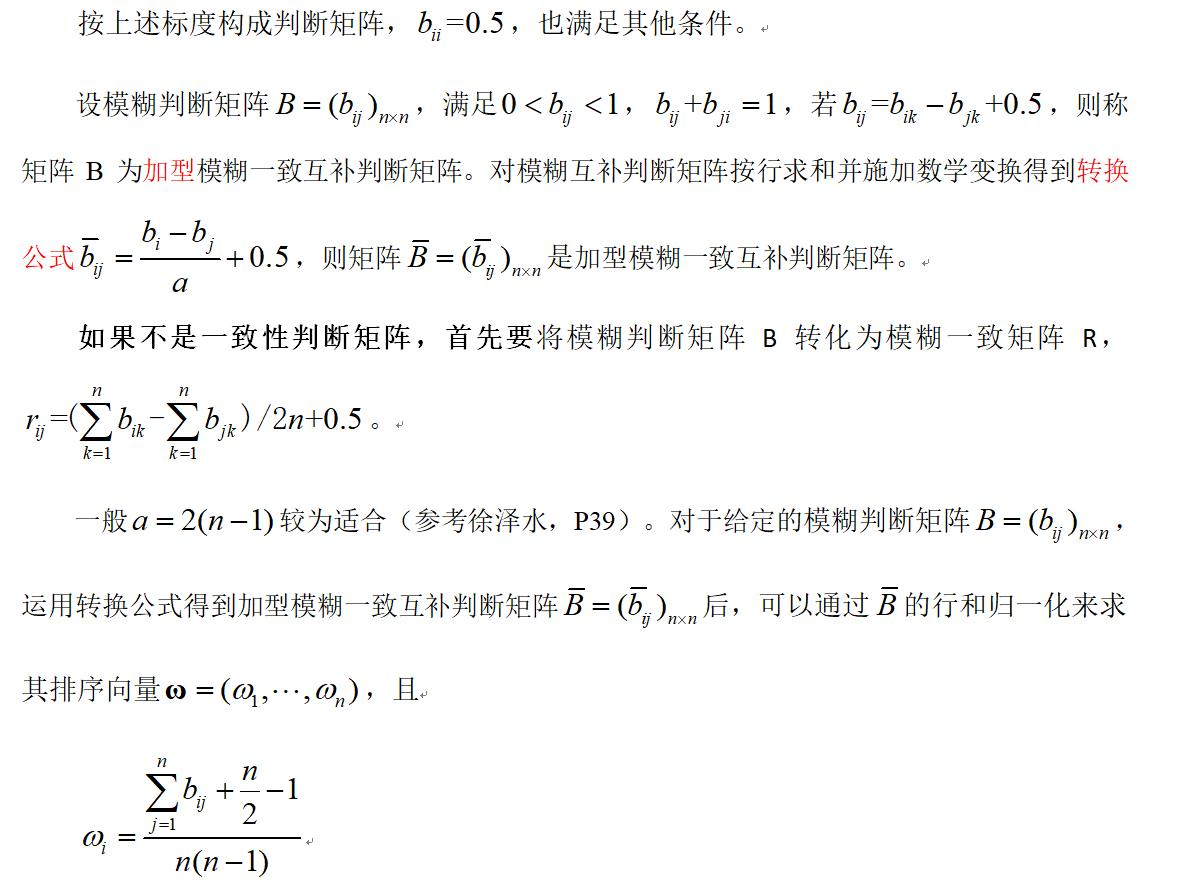此方法称为互补判断矩阵排序的中转法（MTM）。
特点：该方法得到的排序向量的分量之间的差异较小，有时不易区分。
2.乘型互补判断矩阵排序的和积法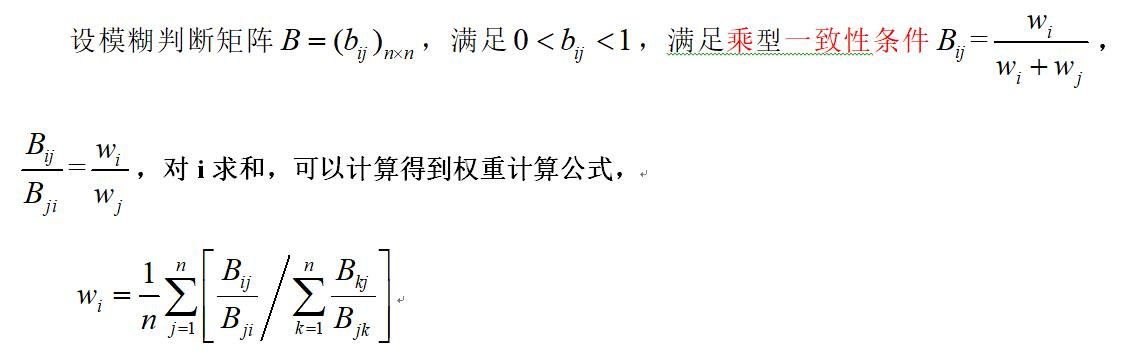特点：该方法得到的排序向量的分量之间的差异较小，有时不易区分。
3.实例
有一规范化后的决策矩阵A，如下表所示，
u1	u2	u3	u4	u5
x1	1	   0.776	0.828	1	0.412
x2	0.516	1	1	0.296	0.480
x3	0.990	0.627	0.669	1	0.508
x4	0.535	0.784	0.970	0.360	0.678
x5	0.788	0.560	0.578	0.589	1
决策者根据0.1-0.9互补标度对属性进行了两两比较，给出互补判断矩阵B。
0.5	0.6	0.5	0.9	0.7
0.4	0.5	0.4	0.7	0.5
0.5	0.6	0.5	0.7	0.2
0.1	0.3	0.3	0.5	0.1
0.3	0.5	0.8	0.9	0.5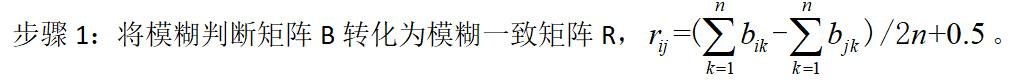步骤2：用互补判断矩阵排序的中转法与和积法计算属性的权重向量。
步骤3：再用属性的权重向量，计算各方案综合属性值
步骤4：对方案进行排序。
程序如下：
clear;clc;
A=[1	    0.776	0.828	1
0.516	1	    1	    0.296
0.990	0.627	0.669	1
0.535	0.784	0.970	0.360
0.788	0.560	0.578	0.589]

B=[0.5	0.6	0.5	0.9	0.7
0.4	0.5	0.4	0.7	0.5
0.5	0.6	0.5	0.7	0.2
0.1	0.3	0.3	0.5	0.1
0.3	0.5	0.8	0.9	0.5]

[m,n]=size(B);
r=sum(B');
for i=1:m
for j=1:n
R(i,j)= (r(i)-r(j))/(2*m)+0.5;
end
end

w1 = (sum(R')+m/2-1)/(m*(m-1));   %中转法

for i=1:n
t=0;
for j=1:n
pp=0;
for k=1:n
pp=pp+R(k,j)/R(j,k);
end
t=t+R(i,j)/R(j,i)/pp;
end
w2(i)=t/n;
end
w2=w2;            %和积法

运行结果：
①	中转法运行结果：w1 =    0.2175    0.2000    0.2000    0.1700    0.2125
②	和积法运行结果：w2=   0.25797	 0.19353	 0.19353	 0.11752	 0.23744
本文是“多属性决策matlab应用”专栏的最后一篇，后面重新开辟两个专栏“模糊多属性决策方法及matlab应用”和“区间数多属性决策方法及matlab应用”。“模糊多属性决策方法及matlab应用”专栏已经发布4篇相关文章。


展开全文matlab 算法 线性代数 动态规划
• ## 权重计算方法

万次阅读 2019-06-10 16:58:47
AHP算法计算层次权重其基本步骤为将问题分解为不同的组成因素，按照因素间的相互关系或者隶属关系将因素按不同因素聚集集合，形成一个多层次的分析结构模型，并由此构建判断（或成对比较）矩阵，依据判断矩阵对层次...
AHP方法
AHP算法计算层次权重其基本步骤为将问题分解为不同的组成因素，按照因素间的相互关系或者隶属关系将因素按不同因素聚集集合，形成一个多层次的分析结构模型，并由此构建判断（或成对比较）矩阵，依据判断矩阵对层次进行单排序并检验是否通过一致性检验，最后计算某一层次所有因素对于最高层(总目标)相对重要性的权值，称为层次总排序。其流程图如图所示：
图# AHP算法计算流程
1.建立层次结构模型
将决策的目标、考虑的因素（决策准则）和决策对象按它们之间的相互关系分为最高层、中间层和最低层，绘出层次结构图。在本例中，决策的目标是计算各分割产品的合理定价，考虑的因素应为市场喜好程度，决策对象为各分割产品。
2.构造判断矩阵
在确定各层次各因素之间的权重时，如果只是定性的结果，则常常不容易被别人接受，因而Santy等人提出一致矩阵法，即不把所有因素放在一起比较，而是两两相互比较，对此时采用相对尺度，以尽可能减少性质不同的诸因素相互比较的困难，以提高准确度。如对某一准则，对其下的各方案进行两两对比，并按其重要性程度评定等级。 为要素 与要素j重要性比较结果。按两两比较结果构成的矩阵称作判断矩阵。判断矩阵具有如下性质：
a_ij=1/a_ji
构造判断矩阵时一般通过两两比对进行确认，一般按照下表比例标度进行确认标度值：
因素i比因素j 量化值
同等重要 1
稍微重要 3
较强重要 5
强烈重要 7
极端重要 9
两相邻判断的中间值 2，4，6，8
3.层次单排序及其一致性检验
对应于判断矩阵最大特征根 的特征向量w，w经归一化后记为W。W的元素为同一层次因素对于上一层次因素某因素相对重要性的排序权值，这一过程称为层次单排序。能否确认层次单排序，则需要进行一致性检验，定义一致性指标为：
CI=  ((λ-n))/(n-1)
式中：λ为判断矩阵特征向量；n为因素数量
CI=0，有完全的一致性；CI 接近于0，有满意的一致性；CI 越大，不一致越严重。为衡量CI的大小，引入随机一致性指标RI：
RI=  (〖CI〗_1+〖CI〗_2+⋯+〖CI〗_n)/n
其中，随机一致性指标RI和判断矩阵的阶数有关，一般情况下，矩阵阶数越大，则出现一致性随机偏离的可能性也越大，本例因素数量为24，查表得RI取值1.6497。
考虑到一致性的偏离可能是由于随机原因造成的，因此在检验判断矩阵是否具有满意的一致性时，还需将CI和随机一致性指标RI进行比较，得出检验系数CR，公式如下：
CR = CI/RI
一般，如果CR<0.1 ，则认为该判断矩阵通过一致性检验，否则就不具有满意一致性。
4.层次总排序及其一致性检验
计算某一层次所有因素对于最高层(总目标)相对重要性的权值，称为层次总排序。
#计算各产品的定价权重
a = [];#a为判断矩阵
n = len(a)
b = []
for i in range(24):
b.append(a/a[i])
B = np.transpose(b)#转置
value,V = np.linalg.eig(B)#value特征值，V为特征向量
value_max = np.argmax(value)#寻找最大的特征值的索引
w1 = V[:,value_max]

for i in w1:
w = w1/sum(i)
W = np.transpose(w) #获得权重矩阵
#一致性检验
CI = (value[value_max]-n)/(n-1)
RI = 1.6793 #查表获得n个阶数下的一致性指标值
CR = CI/RI
if CR < 0.1:
print('通过一致性检验！\n')
else:
print('需重新赋值一致性不被接受！\n')

熵值法
1、基本原理
熵值法是指用来判断某个指标的离散程度的数学方法。离散程度越大，对该指标对综合评价的影响越大。可以用熵值判断某个指标的离散程度。可根据各项指标的变异程度，利用信息熵这个工具，计算出各个指标的权重，为多指标综合评价提供依据。
2、算法步骤
（1）选取n个层次，m个指标，则x_ij为第i个层次的第j个指标的数值；（i=1,2,3…,n;  j = 1,2,3…m)
（2）指标的归一化处理：异质指标的同质化
（3）计算第j项指标下第i个层次占该指标的比重：
（4）计算第j项指标的熵值
（5）计算信息熵冗余度：
（6）计算各项指标的权重值


展开全文• 注：应用程序时，只需要将评判矩阵，也就是判断矩阵，输入到程序中即可，即修改A矩阵，其他地方都不需要修改。  首先进行对矩阵一致性的判断，只有通过一致性检验的矩阵，得到的权重才更可靠，涉及较少变量（如2个...matlab
• function Q=AHP(A) [m,n]=size(A); RI=[0 0 0.58 0.90 1.12 1.24 1.32 1.41 1.45 1.49 1.51]; R=rank(A); %求判断矩阵的秩 [V,D]=eig(A); %求判断矩阵的特征值和特征向量，V特征值，D特征向量；
• 第一讲：层次分析法 模型介绍 评价类问题可用打分解决 根据权重表格计算得分 一个小小的总结 ...判断矩阵计算权重 方法1：算数平均法算权重 方法2：几何平均法求权重 方法3特征值求权重数学建模 层次分析法
• 原始正反矩阵（3X3）n=3： ...1. 计算判断矩阵每一行元素的乘积 0.66 15 0.99 2. 计算每一行的n次方根 0.404 2.466 0.996 sum = 3.866 3. 归一化处理：即获得了每个指标的权重(特征向量) 向量w=[W1', W2',...,Wn']
• 层次分析法 图片来自于：哔哩哔哩 数学建模学习...2.利用判断矩阵计算权重 ω\omegaω， 计算权重的时候，一定要将矩阵 归一化 ！ 一致矩阵可以记为一秩矩阵因为各行各列成比例，因而 rank(A)=1rank(A)=1rank...算法 线性代数
• 一致矩阵的特点:一致矩阵的引理：一致性检验的步骤判断矩阵计算权重算术平均法求权重几何平均法特征值法求权重层次分析法的局限性层次分析法框架图 层次分析法 评价类问题可用打分来解决，也就是说通过分数来量化一...数学建模
• 数学建模一：层次分析法 层次分析法用于解决评价类问题。...3.根据判断矩阵计算权重并计算各方案的权重选择最佳方案实现目标。 例：甲计划外出旅游，备选地点有苏杭、北戴河与桂林。请你确定评价指标、形成评价体系来数学建模
• 判断矩阵进行归一化处理, 得到归一化判断矩阵：c．根据熵的定义，根据各年份评价指标，可以确定评价指标的熵。d．定义熵权。定义了第n个指标的熵后，可得到第n个指标的熵权。f．计算系统的权重值.要求表格形式表格...
• 目前在基于 eCognition 的影像分割过程中波段选择和权重设置较为客观的方法...例如黄汀等(2018)通过波段间的协方差矩阵判断各波段包含信息量的大小，利用波段间的相关矩阵判断各波段间的相关性和信息的冗余度，...
• 引入悲观度指数, 改进和优化区间数层次分析模型, 并通过增加约束条件, 给出了满足一致性逼近要求的区间数判断矩阵的构建过程及矩阵A(x)、半偏差矩阵的计算方法等;通过带有风险偏好信息的区间数互反判断矩阵权重...
• 判断矩阵进行归一化处理, 得到归一化判断矩阵： c．根据熵的定义，根据各年份评价指标，可以确定评价指标的熵。 d．定义熵权。定义了第n个指标的熵后，可得到第n个指标的熵权。 f．计算系统的权重值. 表格整理格式...python
• 在对专家意见进行一致性分析的基础上,集结各位专家给出的属性权重,通过定义备选方案在属性值为实数、区间数和语言值等不同类型属性上的相对优势关系构造判断矩阵,并以此建立方案效用值计算的线性目标规划模型,从而...
• 针对专家判断信息以直觉模糊集给出的直觉模糊群决策矩阵, 提出一种新的客观确定专家权重的方法. 与传统的通过专家评价的差异程度来确定专家权重的思路不同, 该方法通过定义直觉模糊集的模糊熵计算专家判断信息的模糊...
• %此子程序来源参考：《层次分析法在确定企业家评价指标权重中的应用》 function [W,CR]=AHP(P) %计算指标权重 [m,n]=size(P); for i=1:m M_t(i)=prod(P(i,:));...%计算判断矩阵的最大特征值 PW=P*W'; PW_t=Pmatlab
• 通过构建判断矩阵,进行了层次单排序和总排序及对应的一致性检验;最终确定了7大主控因素控制17号煤层底板奥灰突水的权重;以此为基础进行的17号煤层底板奥灰突水脆弱性评价结果与实际吻合效果好。进一步应用基于矩阵...
• ## 模糊评价模型-以2018美赛为例

万次阅读 多人点赞 2019-01-24 01:08:19
文章目录前言什么是模糊数学模型基本步骤模糊评价模型的基本概念模糊集和隶属函数隶属函数的选取隶属矩阵R判断矩阵D根据判断矩阵计算特征权重向量A计算得分检验评价矩阵具体应用确定特征根据原则确定隶属函数计算...数学建模 模糊数学
• 数字电路抽象出来就是有向图，中间的过程是模拟作为边的入度的点使用位计算其值作为该点的权值，最后输入的结果就是类似于计算权重。 步骤： 1 构建图（邻接表、邻接矩阵），【构建的时候，就计算每个点的入度】...点亮数字人生 ccf 拓扑排序
• 借助于粗糙集理论中的属性依赖度定义，构造出属性间相对依赖的客观判断矩阵，在此基础上将该客观判断矩阵和层次分析法中的主观判断矩阵相结合，提出了一种先确定组合判断矩阵，然后通过计算组合判断矩阵权重来进行...层次分析法
• 层次分析法1 建立层次结构模型2 对于同一层次的各元素关于上一层次中某一准则的重要性进行两两比较，构造判断矩阵3 由判断矩阵计算被比较元素对于该准则的相对权重，并进行一致性检验，（检验通过才能够计算权重）a ...数学建模
• 判断矩阵计算相对权重，决定判断矩阵是否近似满足一致性条件，是层次分析法重要组成部分。本文从相对熵的概念出发。试给出一种新的权重计算方法，同时说明，相对熵可以为判断矩阵是否近似满足一致性条件的一个指标...
• 2Floyd算法的构造过程和Warshall算法非常相似，通过初试的权重矩阵，每次加入一个顶点，看这个顶点能否作为中间点来更新图的权重矩阵（以这个点为桥梁，判断是否缩短了原先两点的距离，即Map[...Floyd
• 1.先对判断矩阵进行一致性检验，然后再计算权重。 因为只有通过了一次性检验，权重才有意义。 如果判断矩阵本身就是一个一致矩阵，那么就没有必要进行一致性检验。（即各行各列成倍数关系，就是一个一致矩阵，一般......